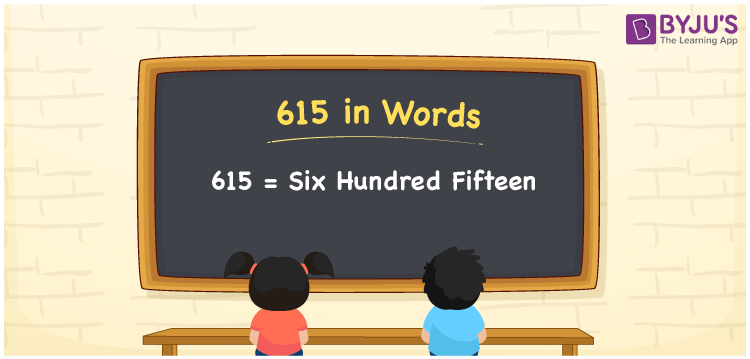# 615 in Words

The number name of 615 is expressed as Six Hundred Fifteen. The number 615 is a three-digit number and its place values help to write the number 615 in words. In both the Indian and the International System of numerals, the number 615 is expressed as six hundred fifteen. This article will guide you to write the procedure of writing the number 615 in words.

 615 in Words: Six Hundred Fifteen. Six Hundred Fifteen in Numerical Form: 615.

## 615 in English Words## How to Write 615 in Words?

The place value table for the number 615 is represented in the below table.

 Hundreds Tens Ones 6 1 5

The expanded form of 615 is as follows:

= 6 × Hundred + 1 × Ten + 5 × One

= 6 × 100 + 1 × 10 + 5 × 1

= 600 + 10 + 5

= 615

= six hundred fifteen

Hence, 615 in words is six hundred fifteen.

615 in words – Six hundred fifteen

Is 615 an odd number? – Yes

Is 615 an even number? – No

Is 615 a perfect square number? – No

Is 615 a perfect cube number? – No

Is 615 a prime number? – No

Is 615 a composite number? – Yes

## Frequently Asked Questions on 615 in Words

Q1

### How to spell 615 in English words?

615 in words is six hundred fifteen.

Q2

### Simplify 600 + 15, and express it in words.

Simplifying 600 + 15, we get 615. Hence, 615 in words is Six hundred fifteen.

Q3

### Is 615 a prime number?

No, 615 is not a prime number.